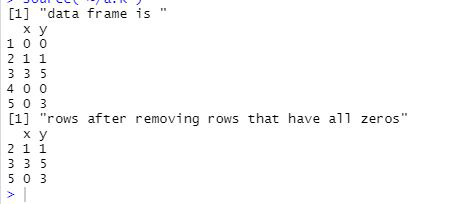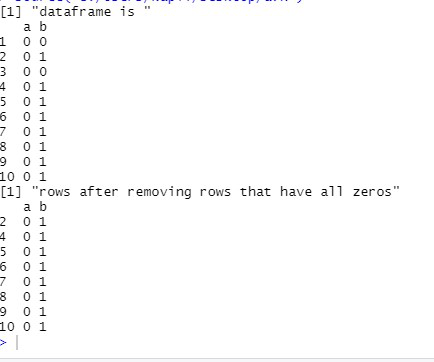Open In App

# How to remove rows that contain all zeros in R dataframe?

In this article, let’s discuss how to rows that contain all zeroes in R dataframe.

Approach:

• Create dataframe
• Get the sum of each row
• Simply remove those rows that have zero-sum. Based on the sum we are getting we will add it to the new dataframe. if the sum is greater than zero then we will add it otherwise not.
• Display dataframe

To calculate the sum of each row rowSums() function can be used.

Syntax:

df[rowSums(df[])>0,]

Where, df is the dataframe.

Example 1:

## R

 `df <- ``data.frame``(x = ``c``(0, 1, 3, 0, 0), y = ``c``(0, 1, 5, 0, 3))`` ` `print``(``"data frame is "``)``print``(df)`` ` `ans = df[``rowSums``(df[])>0,]`` ` `print``(``"rows after removing rows that have all zeros"``)``print``(ans)`

Output:Example 2:

## R

 `a = ``rep``(0,10)``b = ``sample``(``c``(0,1),10,replace=``TRUE``)``df = ``data.frame``(a,b)``print``(``"dataframe is "``)``print``(df)`` ` `ans = df[``rowSums``(df[])>0,]``print``(``"rows after removing rows that have all zeros"``)``print``(ans)`

Output: